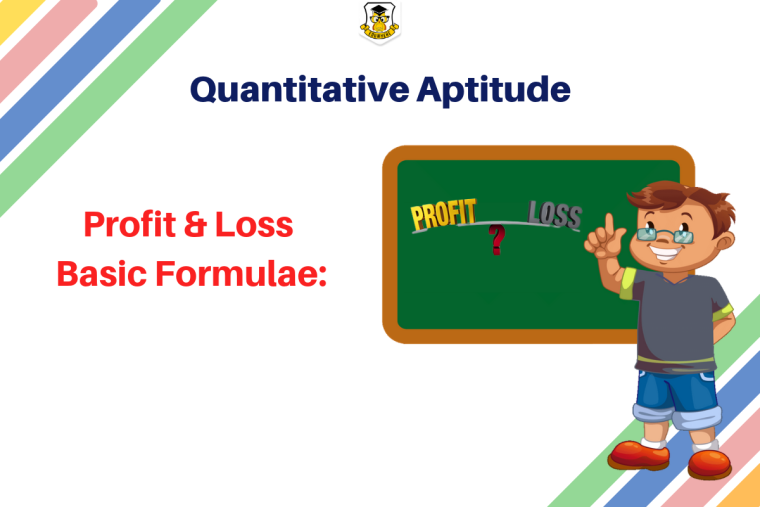## Eduwhere Concept: Profit & LossProfit & Loss plays a key role in the realm of economics and accounting. Questions related to Profit & Loss are likely to appear in your upcoming examination. Here are some basic terms and formulae involved in Profit & Loss.

Profit = S P  – C P

Loss = C P  –  S P

Profit percentage = [Profit/cost price] × 100

Loss percentage = [loss/cost price] × 100

Loss or gain is always calculated over CP.

%Gain = {(Gain*100)/CP}

%Loss = {(Loss*100)/CP}

## Cost Price:

The price at which the article is purchased is called the Cost Price.

Take a look at some important formulae related to Cost Price:

C P = [100/ (100 + gain%)]×S P = [100/ (100 + loss%)]×S P

CP = {100/(100 %Gain)}*SP

CP ={100/(100-%Loss)}*SP

## Selling Price:

The price at which the article is sold to the buyer is called the Selling Price.

S P = (100 +k) % of C P; where profit = k% of C P.

S P = (100 – k)% of C P; where loss = k% of the C P.

S P = [(100 + gain%)/100]×C P = [(100 + loss%)/100]×C P

S P = {(100 %Gain)/100}*CP

S P = {(100-%Loss)/100}*CP

## Listed Price:

The suggested retail price by the manufacturer is referred to as Listed Price.

## Marked Price:

The price on the label of an article/ product is called the marked price. Marked price or List price.

Marked Price = C P + Markup

M P (Marked Price) = C P + (%markup on C P)

When Discount is offered, M.P. > S.P.

When Discount is not offered, M.P. < S.P.

Discount = Marked Price – Selling Price

Prepare for your upcoming competitive exams with Eduwhere!

1

1656